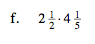### Home > MC2 > Chapter 7 > Lesson 7.1.2 > Problem7-21

7-21.
1. Simplify each expression. Homework Help ✎

1.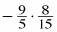2.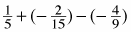3.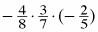4.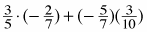5.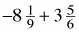6.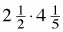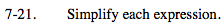Multiply the numerators together and the denominators together.

$-\frac{9(8)}{5(15)} = -\frac{72}{75}$

$-\frac{24}{25}$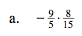Find a common denominator.

$\frac{9}{45}+\left(-\frac{6}{45}\right)-\left(-\frac{20}{45}\right)$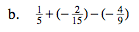Multiply, see (a) for help.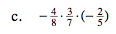Multiply the terms, then find the common denominator.

$-\frac{27}{70}$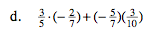Make improper fractions, then find the least common denominator.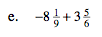$10\frac{1}{2}$

Convert the mixed numbers into improper fractions before multiplying.